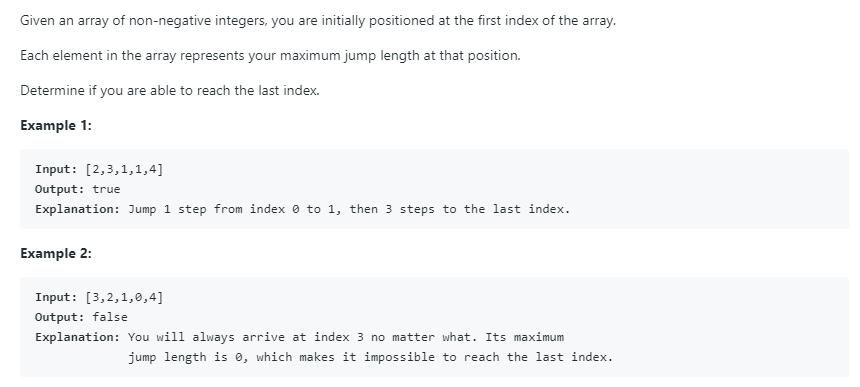# 题目描述（中等难度）45题的时候已经见过这道题了，只不过之前是返回从第 0 个位置能跳到最后一个位置的最小步数，这道题是返回是否能跳过去。

leetCode Solution 中给出的是动态规划的解法，进行了一步一步的优化，但都也比较慢。不过，思路还是值得参考的，上边说的比较详细，这里就不啰嗦了。这里，由于受到 45 题的影响，自己对 45 题的解法改写了一下，从而解决了这个问题。

# 解法一 顺藤摸瓜

45 题的代码。

public int jump(int[] nums) {
int end = 0;
int maxPosition = 0;
int steps = 0;
for(int i = 0; i < nums.length - 1; i++){
//找能跳的最远的
maxPosition = Math.max(maxPosition, nums[i] + i);
if( i == end){ //遇到边界，就更新边界，并且步数加一
end = maxPosition;
steps++;
}
}
return steps;
}


public boolean canJump(int[] nums) {
int end = 0;
int maxPosition = 0;
for(int i = 0; i < nums.length - 1; i++){
//当前更新超过了边界，那么意味着出现了 0 ，直接返回 false
if(end < i){
return false;
}
//找能跳的最远的
maxPosition = Math.max(maxPosition, nums[i] + i);

if( i == end){ //遇到边界，就更新边界，并且步数加一
end = maxPosition;
}
}
//最远的距离是否到答末尾
return maxPosition>=nums.length-1;
}


# 解法二 顺瓜摸藤

public int jump(int[] nums) {
int position = nums.length - 1; //要找的位置
int steps = 0;
while (position != 0) { //是否到了第 0 个位置
for (int i = 0; i < position; i++) {
if (nums[i] >= position - i) {
position = i; //更新要找的位置
steps++;
break;
}
}
}
return steps;
}


public boolean canJump(int[] nums) {
int position = nums.length - 1; //要找的位置
boolean isUpdate = false;
while (position != 0) { //是否到了第 0 个位置
isUpdate = false;
for (int i = 0; i < position; i++) {
if (nums[i] >= position - i) {
position = i; //更新要找的位置
isUpdate = true;
break;
}
}
//如果没有进入 for 循环中的 if 语句，就返回 false
if(!isUpdate){
return false;
}
}
return true;
}


# 解法三

public boolean canJump(int[] nums) {
for (int i = 0; i < nums.length - 1; i++) {
//找到 0 的位置
if (nums[i] == 0) {
int j = i - 1;
boolean isCanSkipZero = false;
while (j >= 0) {
//判断 0 前边的元素能否跳过 0
if (j + nums[j] > i) {
isCanSkipZero = true;
break;
}
j--;
}
if (!isCanSkipZero) {
return false;
}
}
}
return true;
}


public boolean canJump(int[] nums) {
int max = 0;
for (int i = 0; i < nums.length - 1; i++) {
if (nums[i] == 0 && i >= max) {
return false;
}
max = Math.max(max, nums[i] + i);
}
return true;
}


public boolean canJump(int[] nums) {
int max = 0;
for (int i = 0; i < nums.length; i++) {
if (i > max) {
return false;
}
max = Math.max(max, nums[i] + i);
}
return true;
}